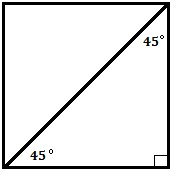Problems
333 - Perimeter II

Perimeter II

Time Limit: 1 sec

The Problem

Suppose you are standing in a Square room. There is a 45 degree angle line is drawn. The Length of the Diagonal is given. Can you tell me what the Perimeter of that room is?

Note: The Perimeter of a Square is 4 * Length of one side of square.The Input

The input file contains a series of line. Each line contains an integer number length of drawn Line of that room, X (X <= 9999). Input is terminated by EOF

The Output

For each line of input the output will show the Perimeter of the room rounded to 2 decimal points.

Sample Input

625

225

12

Sample Output

1767.77

636.40

33.94

Problem Setter: Samia Safa Ahmed